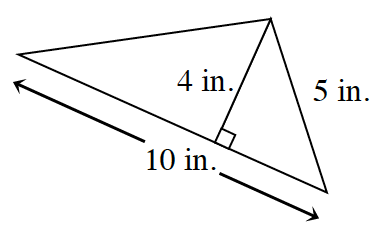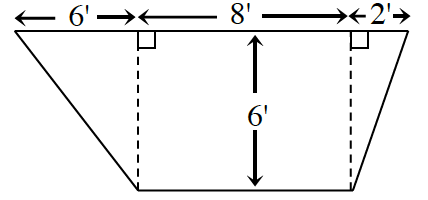### Home > GC > Chapter 2 > Lesson 2.2.4 > Problem2-90

2-90.

Find the area of each figure below. Show all work. Remember to include units in your answer.

Look at the Math Notes box in Lesson 2.2.4 for help on how to find the areas of different shapes.

1. a square:

$49$ square cm

1.1.$\frac{1}{2}(b_{1}+b{_{2}})h$

$\frac{1}{2}(16+8)6\text{ feet}^2$